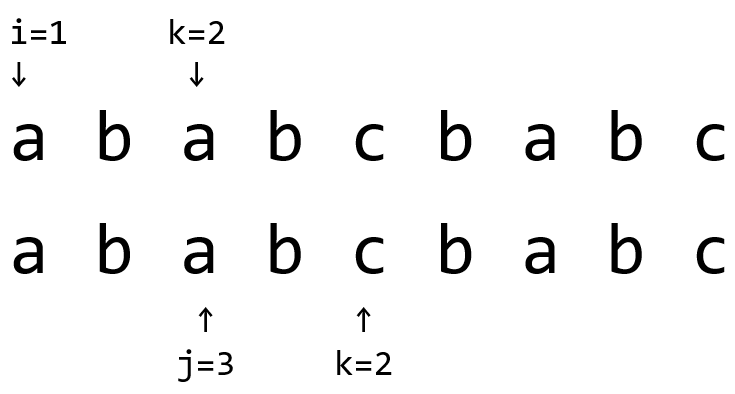# POJ 1509/UVA 719 Glass Beads 题解【字符串】【最小表示法】

算是最小表示法的模板了。

## Description

Once upon a time there was a famous actress. As you may expect, she played mostly Antique Comedies most of all. All the people loved her. But she was not interested in the crowds. Her big hobby were beads of any kind. Many bead makers were working for her and they manufactured new necklaces and bracelets every day. One day she called her main Inspector of Bead Makers (IBM) and told him she wanted a very long and special necklace.

The necklace should be made of glass beads of different sizes connected to each other but without any thread running through the beads, so that means the beads can be disconnected at any point. The actress chose the succession of beads she wants to have and the IBM promised to make the necklace.

But then he realized a problem. The joint between two neighbouring beads is not very robust so it is possible that the necklace will get torn by its own weight. The situation becomes even worse when the necklace is disjoined. Moreover, the point of disconnection is very important. If there are small beads at the beginning, the possibility of tearing is much higher than if there were large beads. IBM wants to test the robustness of a necklace so he needs a program that will be able to determine the worst
possible point of disjoining the beads.

The description of the necklace is a string $A=a_1a_2\dots a_m$ specifying sizes of the particular beads,where the last character am is considered to precede character $a_1$ in circular fashion. The disjoint point $i$ is said to be worse than the disjoint point $j$ if and only if the string $a_ia_{i+1}\cdots a_na_1\dots a_{i−1}$ is lexicografically smaller than the string $a_ja_{j+1}\dots a_na_1\dots a_{j−1}$. String $a_1a_2\dots a_n$ is lexicografically smaller than the string $b_1b_2\dots b_n$ if and only if there exists an integer $i$,$i\le n$, so that $a_j=b_j$ , for each $j$, $1\le j < i$ and $a_i<b_i$.

## Input

The input consists of $N$ cases. The first line of the input contains only positive integer $N$. Then follow  the cases. Each case consists of exactly one line containing necklace description. Maximal length of each description is $10000$ characters. Each bead is represented by a lower-case character of the english alphabet (a–z), where a < b < … < z.

## Output

For each case, print exactly one line containing only one integer — number of the bead which is the first at the worst possible disjoining, i.e. such $i$, that the string $A[i]$ is lexicographically smallest among all the $n$ possible disjoinings of a necklace. If there are more than one solution, print the one with the lowest $i$.

## Sample Input

4
helloworld
amandamanda
dontcallmebfu
aaabaaa

## Sample Output

10
11
6
5

## 题意：

对于一个字符串$S$，定义它的循环同构串为$S_iS_{i+1}\dots S_nS_1\dots S_{i-1},i\in [2,n]$以及它自己。求出循环同构串中字典序最小的一个。

例如字符串helloworld，它的循环同构串有

helloworld
elloworldh
lloworldhe
loworldhel
oworldhell
worldhello
orldhellow
rldhellowo
ldhellowor
dhelloworl

## 题解：

为了找字典序最小的循环同构串，我们可以暴力匹配字典序是否小于当前存的最小串。这样的时间复杂度是$O(n^2)$的，对于$n=10000$的多组数据，当然是过不了的。

事实上求最小表示法有$O(n)$的算法，也许是模板，总之是对两个串各扫描了一遍，时间复杂度上界为$\Theta(2n)$。

首先将字符串复制一遍接在后面，即$S_{n+1}=S_{1},\dots,S_{n+i}=S_{i},\dots,S_{n+n}=S_{n}$，相当于破环为链。接着用两个“指针”，分别指向$i=1,j=2$，开始枚举$k=0,1,2,…,n$比对$S_{i+k}$和$S_{j+k}$，如果$S_{i+k}=S_{j+k}$，没有差异，令k++；如果$S_{i+k}<S_{j+k}$（这里可以直接用char比较大小），说明到$S_{j+k-1}$的前一段都相等，而$S_{i+k}<S_{j+k}$，因此$j\sim j+k-1$这一段不可能成为最小。而前面一段都一样，则最小字典序的串的开头也不会在这一段中，所以还是让$i$这一段来代表最小表示。$S_{i+k}>S_{j+k}$同理。

例如$S_{i+k}<S_{j+k}$，此时因为$i\sim i+k-1$都和$j\sim j+k-1$一样（这两段相等且位置不同，所以进行多的比对并没有意义），所以可以直接跳过前面的段，让$j=j+k+1$。

如果匹配时发现$i=j$那么将其中一个自增即可，就像回到了初始化的状态。这里发现$S_{i+k}<S_{j+k}$时，就可以令$j=j+k+1$，因为$j\sim j+k$都没有更小的（小于a的）字符串串首

实际上在匹配的过程中，设当前最小表示串的串首为$S_t$，我们就是在让所有的$S_{t+k}\ge S_t,k\in \mathbb{N^*}$来让$t=t+k$。同时，也要让所有的$S_{t+k+i}\ge S_{t+i}$，因此在发现两个串有位置不同时就可以跳过。如果最小表示的串首$S_t$被第一次扫描到，那么另一端就一定会被跳过，而同理在满足$S_t$最小时，也在扫描$S_{t+1}$的最小值，以此类推，后面都可以满足，所以可以保证它的正确性，也可以保证输出的值是最小值（大小相同不会更新）。

吐槽：POJ的数据要稍弱于UVA，不小心犯了个错在POJ上还过了……

## Code：

#include<cstdio>
#include<cstring>
char s;
int main()
{
int T;
scanf("%d",&T);
while(T--)
{
scanf("%s",s+1);
int n=strlen(s+1);
for(int i=1;i<=n;++i)
s[i+n]=s[i];
int i,j;
for(i=1,j=2;i<=n&&j<=n;)
for(int k=0;;++k)
{
if(s[i+k]<s[j+k])
{
j=j+k+1;
if(i==j)
++j;
break;
}
else if(s[i+k]>s[j+k])
{
i=i+k+1;
if(i==j)
++i;
break;
}
if(i+k>=2*n)
{
i=n+1;
break;
}
if(j+k>=2*n)
{
j=n+1;
break;
}
}
if(i>n)
printf("%d\n",j);
else
printf("%d\n",i);
}
return 0;
}


### 1 说点什么0 Followers

Most reacted comment
0 Comment authors
Recent comment authors
SubscribewpDiscuz